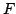Statistica Sinica 18(2008), 1101-1110

ON A CLASS OF DISTRIBUTIONS

WITH SIMPLE EXPONENTIAL TAILS

M. C. Jones

The Open University

Abstract: A general construction is put forward that covers many unimodal univariate distributions with simple, exponentially decaying tails (e.g., asymmetric Laplace, log, and hyperbolic distributions, plus many new models). The proposed family is a subset of a regular exponential family, and many properties flow therefrom. Two main practical points are made in the context of maximum likelihood fitting of these distributions to data. The first is that three, rather than an apparent four, parameters of the distributions suffice. The second is that maximum likelihood estimation of location in the new distributions is equivalent to a standard form of kernel quantile estimation, choice of kernel corresponding to choice of model within the class. This leads to a maximum likelihood method for bandwidth selection in kernel quantile estimation, but with somewhat mixed practical performance.

Key words and phrases: Asymmetric Laplace distribution, bandwidth selection, exponential family, hyperbolic distribution, kernel quantile estimation, log F distribution, maximum likelihood.